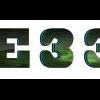Tuts 4 You

# what is work this line## Recommended Postshello every body

i have problem in understand this code:

how work this code in my target :

`movzx eax,byte ptr [edx+eax-1]`

plz illustrate this work

very thanksAs I know, movzx (Zero eXtend) which takes two operands:

Destination: 16- or 32-bit register

Source: 8- or 16-bit register, or 1 byte in memory, or 1 word in memory

The destination must be larger than the source

movzx eax,byte ptr [edx+eax-1]) <=> extends 1-byte value at pointer [edx+eax-1] into eax

•1It takes the byte at address (eax+edx+1) and moves it into Eax, all not affected bytes of eax are set to "00", since you are loading a byte and eax == 4 bytes the result in eax will be something like this : "000000xx"

e.g.

Eax == 12345678

byte ptr ds:[eax+edx+1] == 99h

after "movzx eax,byte ptr [edx+eax-1]" operation:

Eax == 00000099

byte ptr ds:[eax+edx+1] == 99h

•1Can someone give idea too how we can calculate SAR EBX,a?

I beleive for a=1, it returns the value of EBX/2.

how we can get the value for example SAR EBX,3 (EBX = 31323334).

Is it dividing EBX by 2, 3 times?

Best Regards

Edited by E33SAR EAX, 1 ; divide by 2

SAR EAX, 2 ; divide by 4

SAR EAX, 3 ; divide by 8

SAR EAX, 4 ; divide by 16

SAR EAX, 5 ; divide by 32

And so on, it steps like binary.Other shift and rotation instructions

SHL, SHR, ROL, and ROR are the most commonly used shift and rotate instructions. However, there are several others. Let's look at the instructions SAL and SAR.

SAR stands for "Shift Arithmetic Right". SAR is similar to SHR, but SAR preserves the most significant bit. How is this useful?

In Chapter 3, "Binary Manipulations", I very briefly indicated that caution should be used when shifting signed numbers in order to do multiplication or division. Actually, using left-shifts (SHL) to multiply signed numbers by powers of two produces the correct results (as long as there is no overflow or "underflow"). But using right-shifts (SHR) to divide signed numbers by powers of two doesn't work, because the sign bit gets dragged out of the most-significant position. To preserve the sign bit, we can use SAR instead of SHR.

SAR works this way for a single right shift: it saves a copy of the number's sign bit. Then it shifts the number to the right, the same way SHR does. Then it copies the saved sign bit back into the most-significant position. For right shifts by two or more, this procedure is repeated an appropriate number of times.

Let's divide the byte-sized number -20 dec by two, using SAR:

MOV AL, -20 ; AL = -20 dec

SAR AL, 1 ; Signed-right-shift AL by 1

Now let's work out what happens. The two's complement representation of -20 is:

20 dec = 00010100 bin

NOT

-----------------

11101011 bin

+ 1

-----------------

11101100 bin = -20 dec

Now, let's signed-right-shift 11101100 bin:

11101100 bin becomes 11110110 bin

And what does 11110110 bin represent?

11110110 bin

NOT

-----------------

00001001 bin

+ 1

-----------------

00001010 bin = 8 + 2 = 10 dec, so 11110110 bin = -10 dec.

It works: -20 dec divided by two is indeed -10 dec. Note that I have used a byte in this example, but word-sized values work as well

/>http://atrevida.comprenica.com/atrtut16.html

HR,

GhandiIts clear now.

thanks you atom0s & ghandi for these useful replies.